# How to Use MMULT Function in Excel

MMULT function in Excel is used for matrix multiplication. Matrix multiplication is method in Mathematics where 2 matrices gets multiplied to get a new matrix. Here table arrays will act as arrays to function.

Syntax:

=MMULT (array1, array2)

Note: This function works on a condition that the columns of first array must be equals to the rows of the second array. This function will return Value error if the mentioned condition is not fulfilled.

Let’s suppose we have a first table array of 2 x 3 means 2 rows and 3 columns and second array table of 3 x 4 means 3 rows and 4 columns

Condition 1. Columns of first array equal to the rows of second array
Condition 2. The resulting array or matrix will be of 2 x 4 means 2 rows and 4 columns.

Now understand how to use excel MMULT function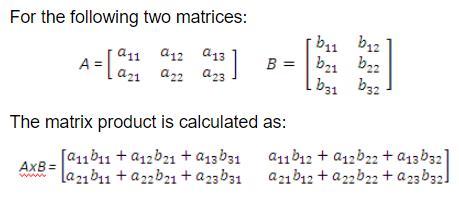Here we have two table arrays of 2 x 2 matrix.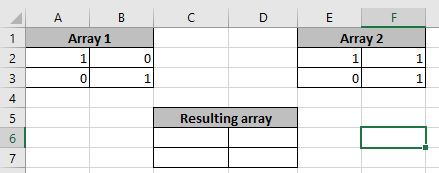Select the size of new table array here which will be 2 x 2.

Use the MMUL excel formula:

{=MMULT(A2:B3, E2:F3)}

Note: Use Ctrl + Shift + Enter to get the result with curly barces and Do not try to put curly braces manually.’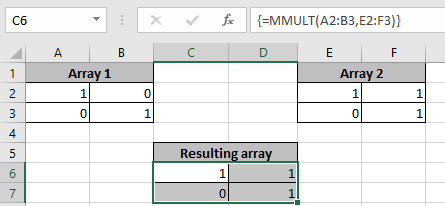As you can see the resulting table array after multiplying two matrices.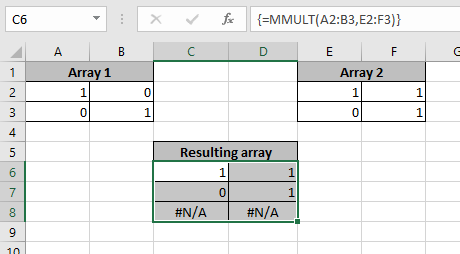The remaining cells generate #NA error if selected array for result is larger than required.

Now take another example for more undrestanding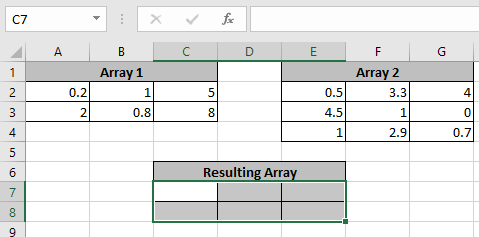Now the resulting array will be of size 2 x 3 mean 2 rows and 3 columns. Select the cells and use the formula to get the product of the 2 arrays

Use the formula

{=MMULT(A2:C3,E2:G4)}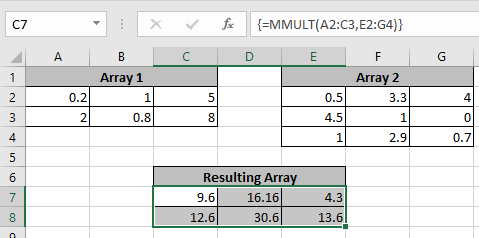As you can see the MMULT function results a new matrix of 2 x 3.

Hope you understood Excel Quartile function. Explore more articles on mathematical Excel functions here. Please state your queries in the comment box below.

Popular Articles:

50 Excel Shortcuts to Increase Your Productivity

How to use the VLOOKUP Function in Excel

How to use the COUNTIF function in Excel 2016

How to Use SUMIF Function in Excel

Terms and Conditions of use

The applications/code on this site are distributed as is and without warranties or liability. In no event shall the owner of the copyrights, or the authors of the applications/code be liable for any loss of profit, any problems or any damage resulting from the use or evaluation of the applications/code.